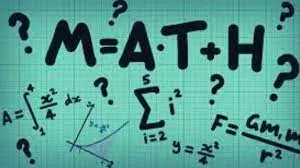# Math riddles: These math riddles are frustratingly challenging. Can you solve them?

Math is a subject that can be super challenging. Can you solve these math riddles?Math is a subject that can be super challenging.  While some give up, many do not give up. Are you one of those who do not give up? Prove it while solving these math riddles.

## Math Riddle 1:

Replace the letter "H" with the math symbols (+, -, x, ÷) so that the following equation holds true.

2 H 1 H 6 H 6 = 48

## Math Riddle 2:

Here comes a sequence-based math problem. Can you find the value of "K" in the sequence?

## Math Riddle 3:

Sophia is a 54-year-old lady.  Sophia's father is an 80-year-old man. Exactly how many years ago was Sophia's father her age?

## Math Riddle 4:

Here comes a tricky math puzzle. There is a huge basket with 12 bananas. You are supposed to divide these 12 bananas among 12 children in such a way that every child gets 1 banana while 1 banana still remains in the basket.

## Math Riddle 5:

Grace was 25 a day before yesterday. The next year Grace will turn 28. The situation is true only one day in a year. On what day does Grace celebrate her birthday?

Math Riddle 1:

Replace the letter "H" with the math symbols (+, -, x, ÷) so that the following equation holds true.

2 H 1 H 6 H 6 = 48

2 x 1 + 6 x 6 = 48

Math Riddle 2:

Here comes a sequence-based math problem. Can you find the value of "K" in the sequence?

.

16 is the value of K.

Math Riddle 3:

Sophia is a 54-year-old lady.  Sophia's father is an 80-year-old man. Exactly how many years ago was Sophia's father her age?

That was 41 years ago. 41 years ago, Sophia was 13 years ago and her father was 39.

Math Riddle 4:

Here comes a tricky math puzzle. There is a huge basket with 12 bananas. You are supposed to divide these 12 bananas among 12 children in such a way that every child gets 1 banana while 1 banana still remains in the basket.

Give all 11 children 1 banana each. Finally, put the last banana in the basket and give the 12th child the basket along with the banana.

Math Riddle 5:

Grace was 25 a day before yesterday. The next year Grace will turn 28. The situation is true only one day in a year. On what day does Grace celebrate her birthday?